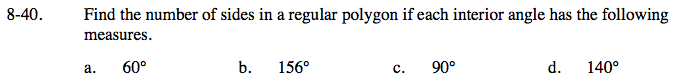### Home > GC > Chapter 8 > Lesson 8.1.4 > Problem8-40

8-40.Refer to the Math Notes box in this lesson to help you find the correct equation to use.

$\frac{180\degree(n-2)}{n}= 60\degree$

180°(n − 2) = 60°n

n = 3

Follow the same steps used in part (a) to solve parts (b), (c), and (d).